Courses

# Maxwell Relations - Thermodynamic and Statistical Physics, CSIR-NET Physical Sciences Physics Notes | EduRev

## Physics for IIT JAM, UGC - NET, CSIR NET

Created by: Akhilesh Thakur

## Physics : Maxwell Relations - Thermodynamic and Statistical Physics, CSIR-NET Physical Sciences Physics Notes | EduRev

The document Maxwell Relations - Thermodynamic and Statistical Physics, CSIR-NET Physical Sciences Physics Notes | EduRev is a part of the Physics Course Physics for IIT JAM, UGC - NET, CSIR NET.
All you need of Physics at this link: Physics

Maxwell equations (thermodynamics)

In thermodynamics, the Maxwell equations are a set of equations derived by application of Euler's reciprocity relation to the thermodynamic characteristic functions. The Maxwell relations, first derived by James Clerk Maxwell, are the following expressions between partial differential quotients: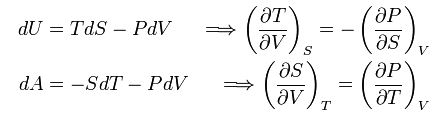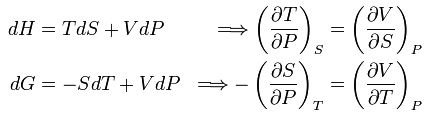The characteristic functions are: U (internal energy), A (Helmholtz free energy), H (enthalpy), and G (Gibbs free energy). The thermodynamic parameters are: T (temperature), S (entropy), P (pressure), and V (volume).

As an example of a derivation, consider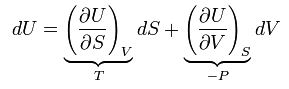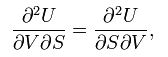which indeed gives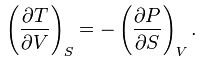159 docs

,

,

,

,

,

,

,

,

,

,

,

,

,

,

,

,

,

,

,

,

,

,

,

,

;